+关注

2019-07(3)

2019-08(105)

2019-09(121)

2019-10(13)

2019-11(5)

# 前言

Seaborn是一个功能强大的Python库，用于增强数据可视化。它为Matplotlib提供了大量高级接口。Seaborn可以很好地处理数据帧，而Matplotlib则不行，它可以让你以更简单的方式绘制引人注目的图表。1. 用Pandas进行数据分析：https://towardsdatascience.com/pandas-for-data-analysis-142be71f63dc
2. 使用Matplotlib进行可视化：https://towardsdatascience.com/visualizations-with-matplotlib-4809394ea223

``````conda install pandas
conda install matplotlib
conda install seaborn``````

``````pip install pandas
pip install matplotlib
pip install seaborn``````

``````import pandas as pd
from matplotlib import pyplot as plt
import seaborn as sns

df = pd.read_csv('Pokemon.csv', index_col = 0, encoding='unicode-escape')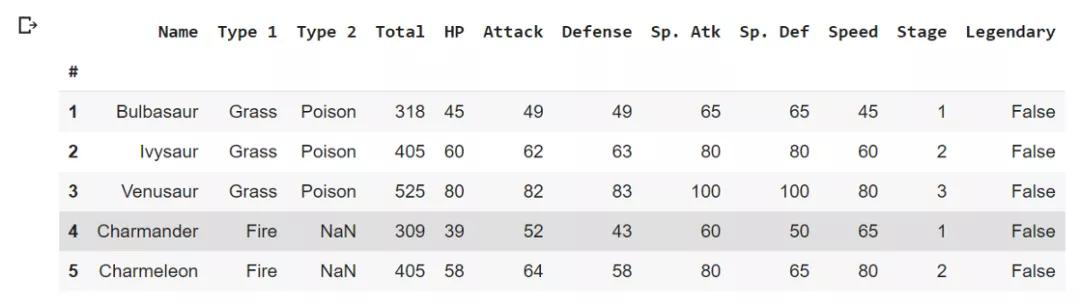# 散点图

``````sns.lmplot(x=’Attack’, y=’Defense’, data=df)
plt.show()``````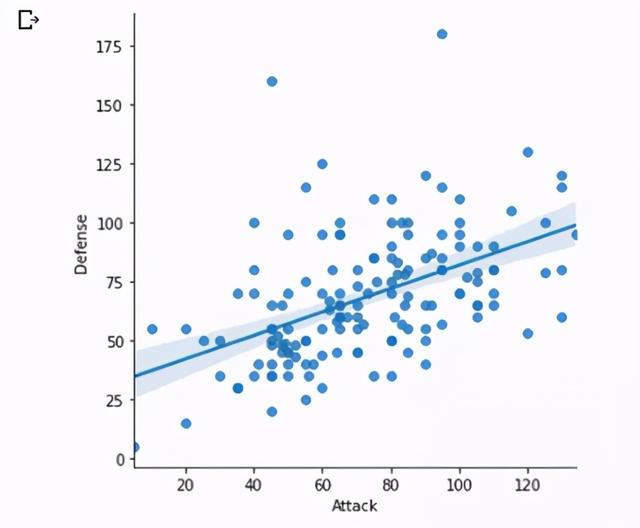``````sns.lmplot(x=’Attack’, y=’Defense’, data=df, fit_reg=False, hue=’Stage’)
plt.show()``````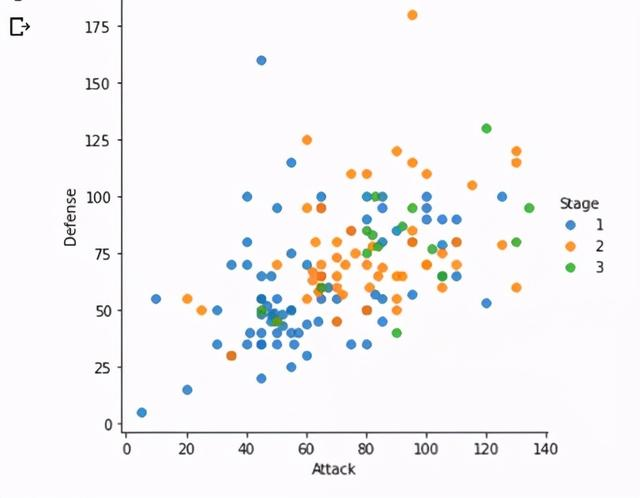# 箱线图

``````df_copy = df.drop([‘Total’, ‘Stage’, ‘Legendary’], axis=1)
sns.boxplot(data=df_copy)``````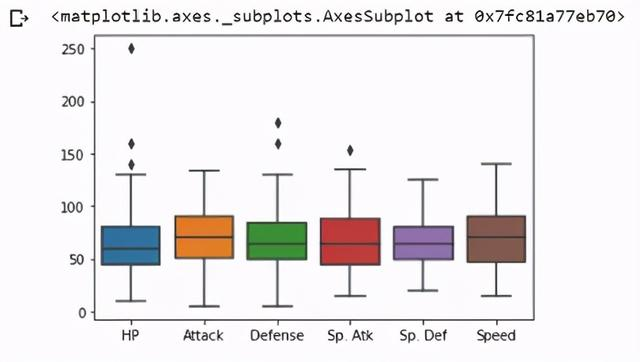# 小提琴图

``````sns.violinplot(data=df_copy)
plt.sow()
``````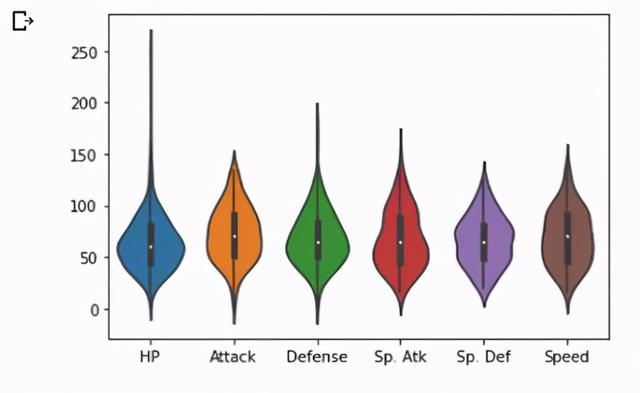``````plt.figure(figsize=(10,6))\sns.violinplot(x='Type 1', y='Attack', data=df)
plt.show()``````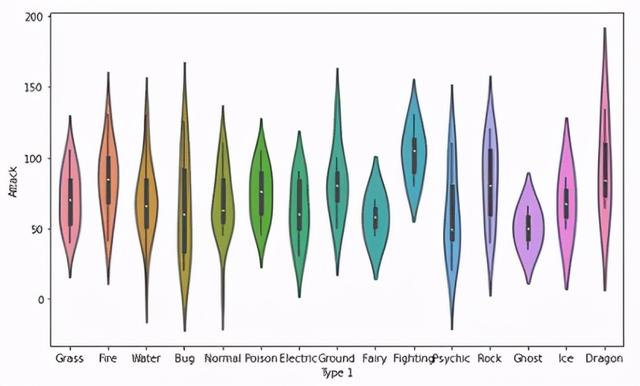# 热图

``````corr = df_copy.corr()
sns.heatmap(corr)``````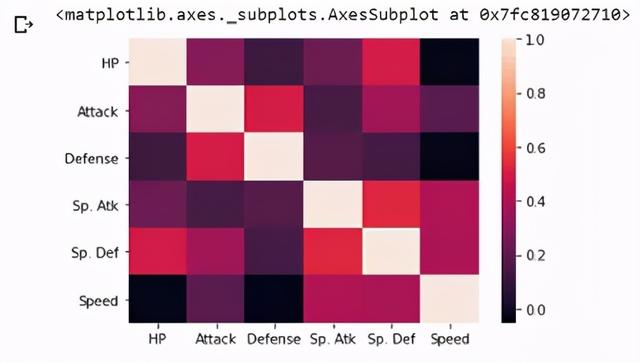# 直方图

``sns.distplot(df.Attack, color=’blue’)``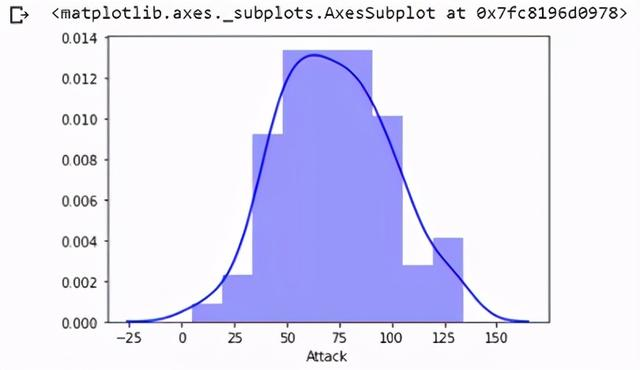# Calplots公司

``````sns.countplot(x=’Type 1', data=df)
plt.xticks(rotation=-45)``````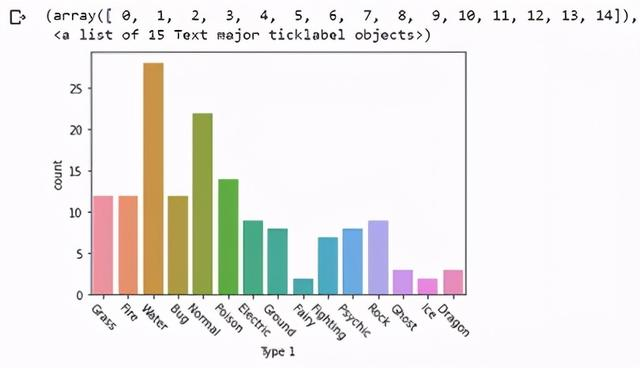# 密度图

``sns.jointplot(df.Attack, df.Defense, kind=’kde’, color=’lightblue’)``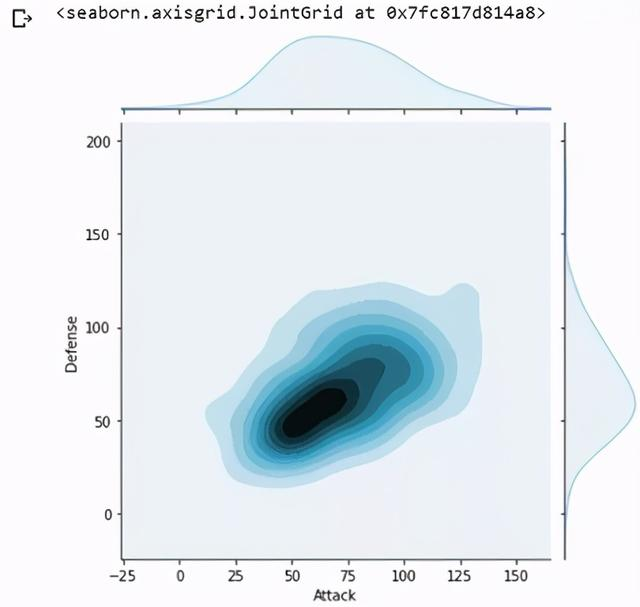“kde”表示我们需要一个密度图。8 0